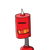# 28) A (-4,2), B (-3,-5), C (3,-2) and D (2, k) are the vertices of a quadrilateral ABCD. If the areaof the quadrilater

28) A (-4,2), B (-3,-5), C (3,-2) and D (2, k) are the vertices of a quadrilateral ABCD. If the area
of the quadrilateral is 28 sq. units, find the value of ‘K’.​

### 2 thoughts on “<br />28) A (-4,2), B (-3,-5), C (3,-2) and D (2, k) are the vertices of a quadrilateral ABCD. If the area<br />of the quadrilater”

1.Let the points be A(4,2),B(3,5),C(3,2) and D(2,3)

The quadrilateral ABCD can be divided into triangles ABC and ACD and hence the

area of the quadrilateral is the sum of the areas of the two triangles.

Area of a triangle with vertices (x

1

,y

1

) ; (x

2

,y

2

) and (x

3

,y

3

) is

2

x

1

(y

2

−y

3

)+x

2

(y

3

−y

1

)+x

3

(y

1

−y

2

)

Hence, substituting the points (x

1

,y

1

)=(−4,−2) ; (x

2

,y

2

)=(−3,−5) and (x

3

,y

3

)=(3,−2) in the area formula, we get

Area of triangle ABC =

2

(−4)(−5+2)+(−3)(−2+2)+3(−2+5)

=

2

12+0+9

=

2

21

=10.5squnits

And, substituting the points (x

1

,y

1

)=(−4,−2) ; (x

2

,y

2

)=(3,−2) and (x

3

,y

3

)=(2,3) in the area formula, we get

Area of triangle ACD =

2

−4(−2−3)+(3)(3+2)+2(−2+2

=

2

20+15+0

=

2

35

=17.5squnits

2.$$\huge \fbox \purple{question}$$

A (-4,2), B (-3,-5), C (3,-2) and D (2, k) are the vertices of a quadrilateral ABCD. If the area

of the quadrilateral is 28 sq. units, find the value of ‘K’.

.

$$\huge \fbox \green{solution}$$

A (-4,2), B (-3,-5), C (3,-2), D(2,k)

Area of triangle ABCD =

$$\frac{1}{2} ( – 4( – 5 + 2) – 3( – 2 + 2) + 3( – 2 + 5)) = \frac{21}{2}$$

sq.unit

Area of Triangle ABC=

$$\frac{1}{2} ( – 4( – 2 – k) + 3(k + 2) + 2( – 2 + 2)) = \frac{(7k + 14)}{2}$$

sq unit

Area of quadrilateral ABCD =28sq. unit

$$therefore \frac{21}{2} + \frac{7k + 14}{2} = 28 \\ \frac{7k + 35}{2} = 28 \\ 7k = 21 \\ therefore \: k = 3$$

hope this helps u..